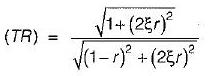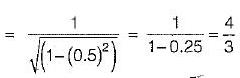Courses

# Test: Linear Vibration Analysis - 3

## 20 Questions MCQ Test Theory of Machines (TOM) | Test: Linear Vibration Analysis - 3

Description
This mock test of Test: Linear Vibration Analysis - 3 for Mechanical Engineering helps you for every Mechanical Engineering entrance exam. This contains 20 Multiple Choice Questions for Mechanical Engineering Test: Linear Vibration Analysis - 3 (mcq) to study with solutions a complete question bank. The solved questions answers in this Test: Linear Vibration Analysis - 3 quiz give you a good mix of easy questions and tough questions. Mechanical Engineering students definitely take this Test: Linear Vibration Analysis - 3 exercise for a better result in the exam. You can find other Test: Linear Vibration Analysis - 3 extra questions, long questions & short questions for Mechanical Engineering on EduRev as well by searching above.
QUESTION: 1

### The ratio of the actual damping coefficient to the critical damping coefficient is called

Solution: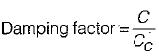QUESTION: 2

Solution:
QUESTION: 3

### If the damping factor for a vibrating system is unity then the system is

Solution: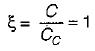It means that system is critically damped and C = Cc

QUESTION: 4

In vibration isolation system, if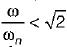the for all values of damping factor, the transmissibility will be

Solution:

In vibration isolation system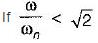Then transmissibility ratio
∈ > 1
This means that the force transmitted to the foundation through elastic support is greater than the force applied.

QUESTION: 5

The ratio of maximum displacement of forced vibration to the deflection due to the static force is known as

Solution:
QUESTION: 6

The normal speed of rotation of a shaft is chosen to be

Solution:
QUESTION: 7

In a multi-rotor system of torsional vibration maximum number of nodes that can occur is

Solution:
QUESTION: 8

A shaft carrying three rotors at ends will have following number of nodes

Solution:

No. of nodes = No. of rotors - 1
= 3 - 1 = 2

QUESTION: 9

During torsional vibration of a shaft, the node is characterized by the

Solution:

Node of a system is a point where amplitude of vibration is zero.

QUESTION: 10

A cantilever beam of negligible weight is carrying a mass M at its free end, and is also resting on an elastic support of stiffness k1, as shown in the figure below.
If k2 represents the bending stiffness of the beam, the natural frequency (rad/s) of the system is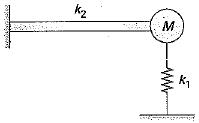Solution:

Natural Frequency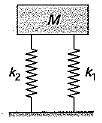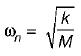where k = k1 + k2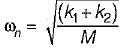QUESTION: 11

A mass of 1 kg is suspended by means of 3 springs as shown in figure. The spring constant K1, K2 and K3 are respectively 1 kN/m, 3 kN/m and 2 kN/m. The natural frequency of the system is approximately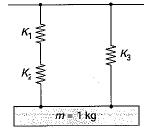Solution: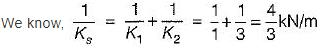Combined stiffness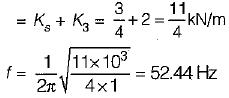QUESTION: 12

The figure shows a critically damped spring-mass system undergoing single degree of freedom vibrations. If m = 5 kg and k = 20 N/m, the value of viscous damping coefficient is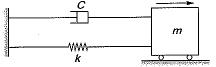Solution: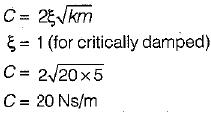QUESTION: 13

Two spring of equal length but having stiffness of 10 N/mm and 15 N/mm support a mass of 2 tonnes in series. Find the frequency of vibration

Solution: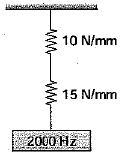In series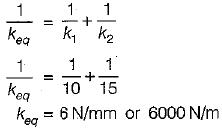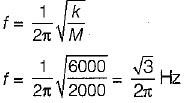QUESTION: 14

Three spring of stiffness k1, k2 and k3 are connected in series. The stiffness of a single spring equivalent to these three springs would be equal to

Solution:

In series combination of springs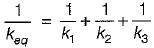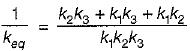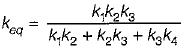QUESTION: 15

Natural frequency of undamped free vibration is 100 rps and damping is provided with damping factor is 0.8 then what is the frequency of damped vibration

Solution: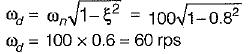QUESTION: 16

An over-damped system

Solution:
QUESTION: 17

Two identical springs of constant K are connected in series and parallel as shown in the figure. A mass m is suspended from them. The ratio of their frequencies of oscillations will be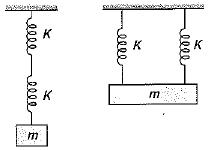Solution: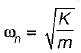For first system Keq = K/2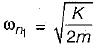For second system Keq = 2K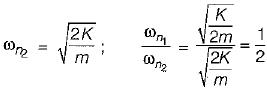QUESTION: 18

The given figure show vibrations of a mass ‘M’ isolated by means of springs and a damper. If an external force ‘F (= A sin ωt) acts on the mass and the damper is not used, then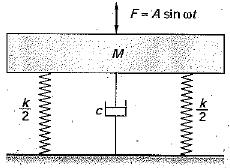Solution: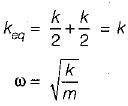QUESTION: 19

A spring-mass suspension has a natural frequency of 40 rad/s. What is the damping ratio required if it is desired to reduce this frequency to 20 rad/s by adding a damper to it?

Solution: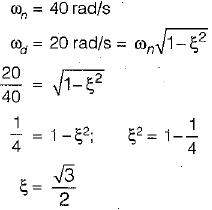QUESTION: 20

A vibrating machine is isolated from the floor using springs, if the ratio of excitation frequency of vibration of machine to the natural frequency of the isolation system is equal to 0.5, the transmissibility ratio of isolation is

Solution:

Given   r  = 0.5
Due to isolation ξ = 0
Transmissibility ratio,